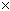SEARCH HOMEMath Central Quandaries & Querieshi my name is admire i am in year 11. i have a little problem i cant form two equations from this question. The cost of producing windscreen wipers blades at a factory at afactory is partly fixed (due to operating overheads ) and partly dependent on the number of blades produced. It costs $300 to produce 1000, and$600 to produce 5000 blades. How much would it cost to produce 24000 blades? (hint: set up two equations and solve the equations)The cost C in dollars is made up of the fixed cost F dollars plus the additional cost that depends on the number of blades produced N. This additional cost is NA where A is the extra cost in dollars to produce one more blade. Thus

C = F + NA

You are told that the cost C is \$300 when the number of blades produced N is 1000, thus

300 = F + 1000A

Use the second fact you are given to produce another equation in the variables F and A and then solve for F and A.

I hope this helps,
PennyMath Central is supported by the University of Regina and The Pacific Institute for the Mathematical Sciences.